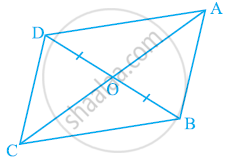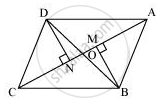# In the given figure, diagonals AC and BD of quadrilateral ABCD intersect at O such that OB = OD. If AB = CD, then show that - Mathematics

In the given figure, diagonals AC and BD of quadrilateral ABCD intersect at O such that OB = OD. If AB = CD, then show that:

(i) ar (DOC) = ar (AOB)

(ii) ar (DCB) = ar (ACB)

(iii) DA || CB or ABCD is a parallelogram.

[Hint: From D and B, draw perpendiculars to AC.]#### SolutionLet us draw DN ⊥ AC and BM ⊥ AC.

(i) In ΔDON and ΔBOM,

∠DNO = ∠BMO (By construction)

∠DON = ∠BOM (Vertically opposite angles)

OD = OB (Given)

By AAS congruence rule,

ΔDON ≅ ΔBOM

∴ DN = BM ... (1)

We know that congruent triangles have equal areas.

∴ Area (ΔDON) = Area (ΔBOM) ... (2)

In ΔDNC and ΔBMA,

∠DNC = ∠BMA (By construction)

CD = AB (Given)

DN = BM [Using equation (1)]

∴ ΔDNC ≅ ΔBMA (RHS congruence rule)

⇒ Area (ΔDNC) = Area (ΔBMA) ... (3)

On adding equations (2) and (3), we obtain

Area (ΔDON) + Area (ΔDNC) = Area (ΔBOM) + Area (ΔBMA)

Therefore, Area (ΔDOC) = Area (ΔAOB)

(ii) We obtained,

Area (ΔDOC) = Area (ΔAOB)

⇒ Area (ΔDOC) + Area (ΔOCB) = Area (ΔAOB) + Area (ΔOCB)

(Adding Area (ΔOCB) to both sides)

⇒ Area (ΔDCB) = Area (ΔACB)

(iii) We obtained,

Area (ΔDCB) = Area (ΔACB)

If two triangles have the same base and equal areas, then these will lie between the same parallels.

∴ DA || CB ... (4)

In ΔDOA and ΔBOC,

∠DOA = ∠BOC (Vertically opposite angles)

OD = OB (Given)

∠ODA = ∠OBC (Alternate opposite angles)

By ASA congruence rule,

ΔDOA ≅ ΔBOC

∴ DA = BC ... (5)

In quadrilateral ABCD, one pair of opposite sides is equal and parallel (AD = BC)

Therefore, ABCD is a parallelogram.

Concept: Corollary: Triangles on the same base and between the same parallels are equal in area.
Is there an error in this question or solution?

#### APPEARS IN

NCERT Class 9 Maths
Chapter 9 Areas of Parallelograms and Triangles
Exercise 9.3 | Q 6 | Page 163